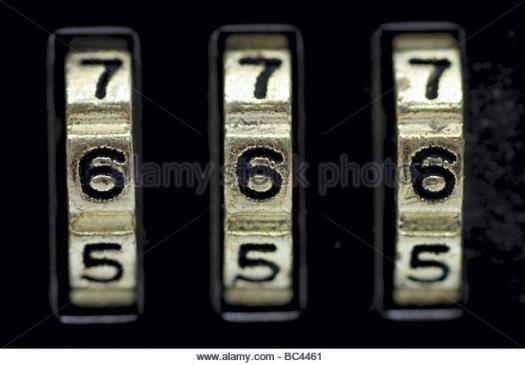# What Do You Know About Solving Equations?

10 Questions | Total Attempts: 115SettingsWhat do you know about solving equations? The process of solving equations of various forms. It also shows you how to check your answer three different ways: algebraically, graphically, and using the concept of equivalence.

• 1.
In ...., an equation is a statement of an equality containing one or more variables.
• A.

Literature

• B.

Mathematics

• C.

Biology

• D.

Chemistry

• 2.
..... are also called unknowns.
• A.

Variables

• B.

Constants

• C.

Operators

• D.

Coefficients

• 3.
There are how many kinds of equations?
• A.

One

• B.

Two

• C.

Three

• D.

Four

• 4.
An equation is written as ....
• A.

One expression

• B.

Three expressions

• C.

Two expressions

• D.

Four expressions

• 5.
An equation is written as two expressions, connected by a .....
• A.

Equal sign

• B.

• C.

Multiplication sign

• D.

Subtraction sign

• 6.
Algebra studies how many main families of equations?
• A.

Four

• B.

Three

• C.

One

• D.

Two

• 7.
The "=" symbol, which appears in every equation, was invented in ....
• A.

1557

• B.

1616

• C.

1558

• D.

1996

• 8.
The "=" symbol, which appears in every equation, was invented in 1557 by .....
• A.

Albert Eisntein

• B.

Robert Recorde

• C.

Isaac Newton

• D.

Bill Nye

• 9.
Mathematics is usually studied in ....
• A.

Home

• B.

School

• C.

Museum

• D.

Restaurant

• 10.
Who is not known as a mathematician here?
• A.

Albert Einstein

• B.

Isaac Newton

• C.

Will Smith

• D.

Pythagoras

Related TopicsBack to top